sk|cz|

# Arithmetic Progression

1.Characterize an aritmetic progression:

A progression (a2)n+1 is told to be „arithmetic“ if and only if exists such d є R real number, so that for all    n є N stands  an+1 = an + d
Number d is called arithmetic progression difference.

For an arithmetic progression stands:

• an = a1 + (n-1)d
• ar = as + (r-s)d
• s = a1 + a2 + a3 + ... + an =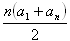• If d > 0 the progression is increasing
• If d < 0 the progression is decreasing
• If d = 0 the progression is constant

2.Find out whether the following progression is arithmetic: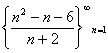Solution:
a1 = -2, a2 = -1, a3 = 0, a4 = 1, a5 = 2  etc.

The progression is arithmetic;  difference d = 1.

3.Enumerate first 6 progression members of an arithmetic progression that fits following conditions:

a1 + a4 + a6 = 71
a5 – a2 – a3 = 2
Solution:
a1 + a1 +3d +a1 +5d = 71
a1 + 4d – a1-d –a1 -2d = 2

3a1 + 8d = 71
-a1 + d =2/3

3a1 + 8d = 71
-3a1 + 3d = 6

a1 – d = -2
a1 = d - 2
a1 = 7 - 2
a1 = 5

11d = 77
d = 7

(a2)n-16 = 5;12;19;26;33;40

4.Enumerate first 6 progression members of an arithmetic progression that fits following conditions: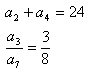Solution: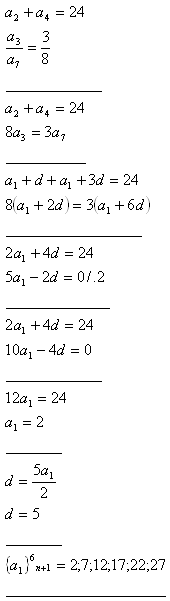5.An aritmetic progression consists of 8 numbers. Sum of the middle members is 41, sum of the first and the last member is 114. Enumerate the progression members.

Solution: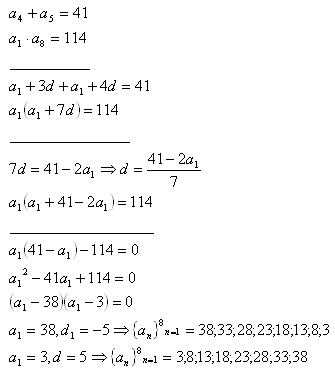6.Insert 4 numbers between the roots of the quadratic equation x2 – 16x +39 = 0 so that they will make an arithmetic progression.

Solution: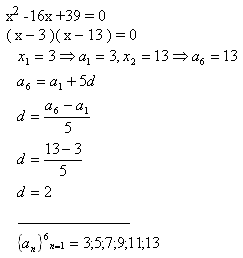Numbers to insert: 5;7;9;11

7.The lengths of the sides of a right triangle make an arithmetic progression. The longer cathetus is 24 cm long. Find out the perimeter of the triangle.

Solution: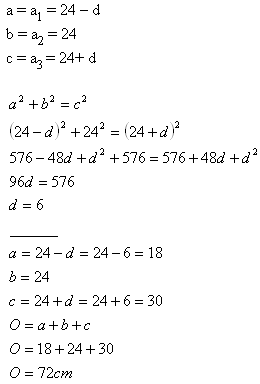The perimeter of the triangle equals 72 cm.

8.Find out the sum of integers 1+2+3+4+5+6+7+.............+100.

Solution: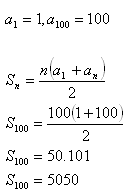Sum of the numbers S = 5050.

9.Iron pipes are stored in 8 rows. The top row contains of 13 pipes, next row always contains of one more pipe than the previous one. How many pipes are there?

Solution: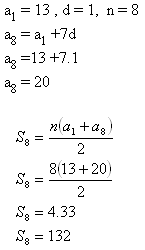There are 132 pipes.

10.The angles in a triangle make an arithmetic progression. The smallest angle is 20°. Determine sizes of the other angles.

Solution: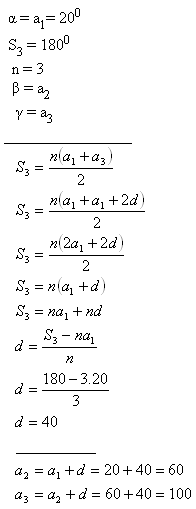The angles are 20°, 60° and 100°.

11.Sizes of the sides of a cuboid make 3 members of an arithmetic progression. Sum of the sizes equals 24 cm, the volume of the cuboid equals 312 cm3. Determine the sizes of the sides.

Solution: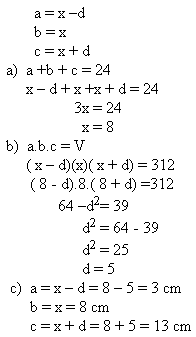The sizes of the sides are 3 cm, 8 cm and 13cm.

12.How long would a rock be falling to a mine somewhere in South America which is deep 2500 m if it moves 4,904 m in the first second and extra 9,808 m for every next second?

Solution: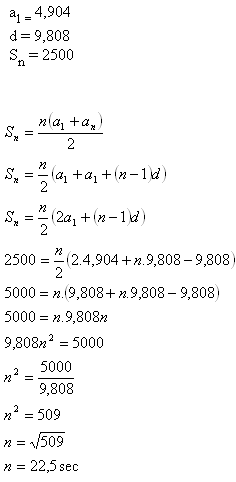The rock would fall for approximately 22,5 sec.